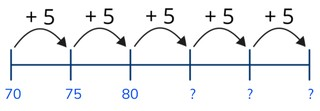Skip counting by fives to 100

# Skip counting by fives to 100

Skip count by fives to 100

No account needed.8,000 schools use Gynzy92,000 teachers use Gynzy1,600,000 students use Gynzy

## General

Students learn to skip count by fives to 100.

## Standards

CCSS.Math.Content.2.NBT.A.2

## Learning objective

Students will be able to skip count by fives to 100.

## Introduction

Show the selection of pencils on the interactive whiteboard. Ask students how many pencils they think there are. Show that there are 40 pencils. Also explain that it is easier to count them in groups of five.

## Instruction

Explain to students that multiples of five are numbers that end with a 5 or a 0 in the ones place. By knowing this, you can easily skip count by fives. While skip counting by fives, you count from a number ending with a 0 to a number ending with a 5. Show the number sequence with the numbers 0 to 50 and have the students count along with you as you go through the row. Point at the numbers as you count. Then show the number line from 55 to 100 and ask the students to count along with you as you point at the numbers. Show a number line in which the jumps of five are shown. Ask students which numbers belong at the question marks. Then ask students to skip count by fives. Ask them to take 3 jumps of five from the number given on the interactive whiteboard. Ask them what number the end on and have them drag the numbers to the number line. Then explain to students that you can also skip count backward by fives. Point at the numbers and count backward with the students from 100 to 0 skip counting by fives. Show a number line with a few question marks. Ask students to determine which numbers belong at the question marks? As a class, practice skip counting backward and complete the number line. Then have students practice skip counting forward and backward by fives and have them write the missing numbers on a sheet of paper or mini whiteboard. Have them hold up their answers to check their work.

Check that students are able to skip count by fives to 100 by asking the following questions:
- What are multiples of five?
- What are multiples of ten?
- How do you skip count by fives?
- Skip count forward by fives from 35 to 100.
- Skip count backward by fives from 70 to 0.

## Quiz

Students are given a selection of exercises in which they are asked to skip count forward and backward by fives. They are first given support with a number line, and then are asked to independently skip count.

## Closing

Discuss with students that it is important to be able to skip count by fives so that you can easily determine how many you have and can work with larger numbers. Ask students to find the missing numbers. Once complete, use the missing numbers to complete the blank number sequence matching colors. Then ask a student to come to the front of the class and show then a number. They must jump to that number. Each jump is five. The student must make the correct number of jumps and their classmates must guess which number they jumped to. Repeat this with jumping backward.

## Teaching tips

Students who have difficulty with this learning goal can be supported by making use of the number line. Have students follow the jumps on the number line, or by counting one by one to check the jumps they make. Remind them that when skip counting in fives, the number goes from a 0 to a 5 in the ones place each time.

## Instruction materials

Optional: Number cards to 100 in multiples of 5

### The online teaching platform for interactive whiteboards and displays in schools

• Save time building lessons

• Manage the classroom more efficiently

• Increase student engagement